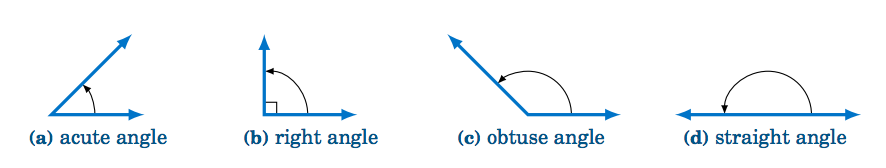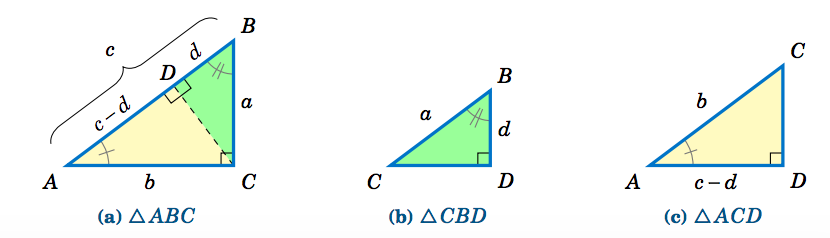$$\newcommand{\id}{\mathrm{id}}$$ $$\newcommand{\Span}{\mathrm{span}}$$ $$\newcommand{\kernel}{\mathrm{null}\,}$$ $$\newcommand{\range}{\mathrm{range}\,}$$ $$\newcommand{\RealPart}{\mathrm{Re}}$$ $$\newcommand{\ImaginaryPart}{\mathrm{Im}}$$ $$\newcommand{\Argument}{\mathrm{Arg}}$$ $$\newcommand{\norm}{\| #1 \|}$$ $$\newcommand{\inner}{\langle #1, #2 \rangle}$$ $$\newcommand{\Span}{\mathrm{span}}$$

1.1: Angles

•• Contributed by Michael Corral
• Professor (Mathematics) at Schoolcraft College

$$\newcommand{\vecs}{\overset { \rightharpoonup} {\mathbf{#1}} }$$

$$\newcommand{\vecd}{\overset{-\!-\!\rightharpoonup}{\vphantom{a}\smash {#1}}}$$

Recall the following definitions from elementary geometry:

1. An angle is acute if it is between $$0°$$ and $$90°$$.
2. An angle is a right angle if it equals $$90°$$.
3. An angle is obtuse if it is between $$90°$$ and $$180°$$.
4. An angle is a straight angle if it equals $$180°$$.Figure 1.1.1 Types of angles

In elementary geometry, angles are always considered to be positive and not larger than $$360^\circ$$. For now we will only consider such angles. The following definitions will be used throughout the text:

1. Two acute angles are complementary if their sum equals $$90^◦$$ . In other words, if $$0^◦ ≤ ∠ A , ∠B ≤ 90^◦ \text{ then }∠ A \text{ and }∠B$$ are complementary if $$∠ A +∠B = 90^◦$$ .
2. Two angles between $$0^◦ \text{ and }180^◦$$ are supplementary if their sum equals $$180^◦$$ . In other words, if $$0^◦ ≤ ∠ A , ∠B ≤ 180^◦ \text{ then }∠ A \text{ and }∠B$$ are supplementary if $$∠ A +∠B = 180^◦$$ .
3. Two angles between $$0^◦ \text{ and }360^◦$$ are conjugate (or explementary) if their sum equals $$360^◦$$ . In other words, if $$0^◦ ≤ ∠ A , ∠B ≤ 360^◦ \text{ then }∠ A \text{ and }∠B\text{ are conjugate if }∠ A+∠B = 360^◦$$ .Figure 1.1.2 Types of pairs of angles

Instead of using the angle notation $$∠ A$$ to denote an angle, we will sometimes use just a capital letter by itself (e.g. $$A, B, C$$) or a lowercase variable name (e.g. $$x, y, t$$). It is also common to use letters (either uppercase or lowercase) from the Greek alphabet, shown in the table below, to represent angles:

Table 1.1 The Greek alphabetIn elementary geometry you learned that the sum of the angles in a triangle equals $$180^◦$$, and that an isosceles triangle is a triangle with two sides of equal length. Recall that in a right triangle one of the angles is a right angle. Thus, in a right triangle one of the angles is $$90^◦$$ and the other two angles are acute angles whose sum is $$90^◦$$ (i.e. the other two angles are complementary angles).

Example 1.1

For each triangle below, determine the unknown angle(s):Note: We will sometimes refer to the angles of a triangle by their vertex points. For example, in the first triangle above we will simply refer to the angle $$\angle\,BAC$$ as angle $$A$$.

Solution:

For triangle $$\triangle\,ABC$$, $$A = 35^\circ$$ and $$C = 20^\circ$$, and we know that $$A + B + C = 180^\circ$$, so

$\nonumber 35^◦ + B + 20^◦ = 180^◦ ⇒ B = 180^◦ − 35^◦ − 20^◦ ⇒ \fbox{$$B = 125^◦$$} .$

For the right triangle $$△DEF,\, E = 53^◦ \text{ and }F = 90^◦$$ , and we know that the two acute angles $$D$$ and $$E$$ are complementary, so

$\nonumber D + E = 90^◦ ⇒ D = 90^◦ − 53^◦ ⇒ \fbox{$$D = 37^◦$$} .$

For triangle $$△ XY Z$$, the angles are in terms of an unknown number $$α$$, but we do know that $$X +Y + Z = 180^◦$$ , which we can use to solve for $$α$$ and then use that to solve for $$X, Y, \text{ and }Z$$:

$\nonumber α + 3α + α = 180^◦ ⇒ 5α = 180^◦ ⇒ α = 36^◦ ⇒ \fbox{$$X = 36^◦ ,\, Y = 3×36^◦ = 108^◦ ,\, Z = 36^◦$$}$

Example 1.2: Thales' Theorem

Thales' Theorem states that if $$A, \, B,\text{ and }C$$ are (distinct) points on a circle such that the line segment $$\overline{AB}$$ is a diameter of the circle, then the angle $$\angle\,ACB$$ is a right angle (see Figure 1.1.3(a)). In other words, the triangle $$\triangle\,ABC$$ is a right triangle.Figure 1.1.3 Thales’ Theorem: $$∠ ACB = 90^◦$$

To prove this, let $$O$$ be the center of the circle and draw the line segment $$\overline{OC}$$, as in Figure 1.1.3(b). Let $$α = ∠BAC \text{ and }β = ∠ ABC$$. Since $$\overline{AB}$$ is a diameter of the circle, $$\overline{OA} \text{ and }\overline{OC}$$ have the same length (namely, the circle’s radius). This means that $$△OAC \text{ is an isosceles triangle, and so }∠OCA = ∠OAC = α$$. Likewise, $$△OBC$$ is an isosceles triangle and $$∠OCB = ∠OBC = β$$. So we see that $$∠ ACB = α+β$$. And since the angles of $$△ ABC$$ must add up to $$180^◦$$ , we see that $$180^◦ = α+(α+β)+β = 2 (α+β), \text{ so }α+β = 90^◦$$ . Thus, $$∠ ACB = 90^◦$$ . QED

By knowing the lengths of two sides of a right triangle, the length of the third side can be determined by using the Pythagorean Theorem:

Theorem 1.1. Pythagorean Theorem

The square of the length of the hypotenuse of a right triangle is equal to the sum of the squares of the lengths of its legs.Figure 1.1.5 Similar triangles $$△ ABC, △CBD, △ ACD$$

Recall that triangles are similar if their corresponding angles are equal, and that similarity implies that corresponding sides are proportional. Thus, since $$\triangle\,ABC$$ is similar to $$\triangle\,CBD$$, by proportionality of corresponding sides we see that

$\nonumber \overline{AB}~\text{is to}~\overline{CB}~\text{(hypotenuses)}\text{ as } \overline{BC}~\text{is to}~\overline{BD}~\text{(vertical legs)} \quad\Rightarrow\quad \frac{c}{a} ~=~ \frac{a}{d} \quad\Rightarrow\quad cd ~=~ a^2 ~.$

Since $$\triangle\,ABC$$ is similar to $$\triangle\,ACD$$, comparing horizontal legs and hypotenuses gives

$\nonumber \frac{b}{c-d} ~=~ \frac{c}{b} \quad\Rightarrow\quad b^2 ~=~ c^2 ~-~ cd ~=~ c ^2 ~-~ a^2 \quad\Rightarrow\quad a^2 ~+~ b^2 ~=~ c^2 ~. \textbf{QED}$

Note: The symbols $$\perp$$ and $$\sim$$ denote perpendicularity and similarity, respectively. For example, in the above proof we had $$\,\overline{CD} \perp \overline{AB}\,$$ and $$\,\triangle\,ABC \sim \triangle\,CBD \sim \triangle\,ACD$$.

Example 1.3

For each right triangle below, determine the length of the unknown side:Solution:

For triangle $$\triangle\,ABC$$, the Pythagorean Theorem says that

$\nonumber a^2 ~+~ 4^2 ~=~ 5^2 \quad\Rightarrow\quad a^2 ~=~ 25 ~-~ 16 ~=~ 9 \quad\Rightarrow\quad \fbox{$$a ~=~ 3$$} ~.$

For triangle $$\triangle\,DEF$$, the Pythagorean Theorem says that

$\nonumber e^2 ~+~ 1^2 ~=~ 2^2 \quad\Rightarrow\quad e^2 ~=~ 4 ~-~ 1 ~=~ 3 \quad\Rightarrow\quad \fbox{e ~=~ \sqrt{3}} ~.$

For triangle $$\triangle\,XYZ$$, the Pythagorean Theorem says that

$\nonumber 1^2 ~+~ 1^2 ~=~ z^2 \quad\Rightarrow\quad z^2 ~=~ 2 \quad\Rightarrow\quad \fbox{z ~=~ \sqrt{2}} ~.$

Example 1.4

A 17 ft ladder leaning against a wall has its foot 8 ft from the base of the wall. At what height is the top of the ladder touching the wall?Solution:

Let $$h$$ be the height at which the ladder touches the wall. We can assume that the ground makes a right angle with the wall, as in the picture on the right. Then we see that the ladder, ground, and wall form a right triangle with a hypotenuse of length 17 ft (the length of the ladder) and legs with lengths 8 ft and $$h$$ ft. So by the Pythagorean Theorem, we have

$\nonumber h^2 ~+~ 8^2 ~=~ 17^2 \quad\Rightarrow\quad h^2 ~=~ 289 ~-~ 64 ~=~ 225 \quad\Rightarrow\quad \fbox{h ~=~ 15 ~\text{ft}} ~.$